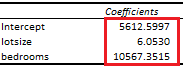# Multiple Linear Regression

Last Update: February 21, 2022

Multiple linear regression is used to model linear relationship between one dependent or explained variable  and two or more independent or explanatory variables . Variable  is also known as target or response feature and variables  are also known as predictor features.

As example, we can fit a three-variable multiple linear regression model with formula . Notice that we are using ˄ or hat character in formula notation because they are estimates. Regression fitted values  are the estimated  values. Estimated constant coefficient  is the  value when  and . Estimated partial regression coefficient  is the estimated change in  when  changes in one unit while holding  constant. Similarly, estimated partial regression coefficient  is the estimated change in  when  changes in one unit while holding  constant.

Model fitting can be done using ordinary least squares method with formula . This method minimizes the sum of squared regression residuals . Regression residuals  with formula  are the estimated differences between actual  and fitted  values.

Below, we find an example of estimated coefficients from multiple linear regression of house price explained by its lot size and number of bedrooms .Table 1. Microsoft Excel® estimated coefficients from multiple linear regression of house price explained by its lot size and number of bedrooms.

Courses

My online courses are hosted at Teachable website.

For more details on this concept, you can view my Linear Regression Courses.

 Data Description: Sales prices of houses sold in the city of Windsor, Canada, during July, August and September, 1987.

Original Source: Anglin, P., and Gencay, R. (1996). Semiparametric Estimation of a Hedonic Price Function. Journal of Applied Econometrics, 11, 633–648.

Source: AER R Package HousePrices Object. Christian Kleiber and Achim Zeileis. (2008). Applied Econometrics with R. Springer-Verlag, New York.

+